# MOSFET Basics

I've seen a lot of questions recently on various forums involving MOSFET projects not working as expected.
I've been meaning to write up a quick summary of how to use them for simple microcontroller projects, so I figured this was a good time to do it.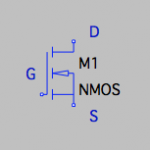This is the symbol for a MOSFET. Specifically an N-channel, enhancement mode MOSFET – the most commonly used type. There are also P-channel and depletion mode MOSFETs all of which operate differently from what I'm about to describe.

MOSFETs are generally used as switches in electronic circuits. They have three terminals:

• Gate – the control input
• Drain – the switch input
• Source – the switch output

There are also a couple of specifications that are important for MOSFETs at this point.

• VDS – the maximum voltage from drain to source.
• ID – the maximum current entering the drain terminal.
• rDS(on) – the resistance of the device when fully switched on.
• VGS(th) – the gate to source threshold voltage – the voltage where the device starts to turn on.

Now that that's out of the way, let's talk about how they actually work.
With no voltage on the gate terminal, there is no current allowed to flow between the drain and source terminals. In fact, anywhere below the threshold voltage there will be no current. Once the threshold has been reached, then a small amount of current begins to flow. The resistance of the MOSFET is very high at this point, so it dominates the circuit resistance. As more and more gate voltage is applied, the resistance of the device decreases until the device is fully on. Here, the MOSFET resistance will be very close to rDS(on), and typically this is going to be a fraction of an ohm.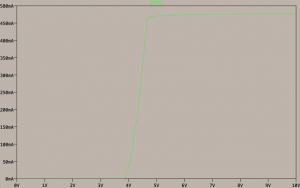Looking at this graph, the drain current is plotted as a function of the gate voltage, for one particular MOSFET and load combination. From 0 to nearly 4 volts, no current is allowed to pass. The threshold voltage is approximately 3.8 V in this case. Once that voltage is reached, as the gate voltage increases, the current also increases. Once the device is passing as much current as the circuit needs, additional gate voltage doesn't produce an effect.

Naively, when people think about a switch, they tend to want to switch the positive side of the circuit. The voltage to switch is tied to the drain, the load (represented by the resistor) is attached to the source, and a voltage higher than the threshold is applied to the gate. That produces a setup like this.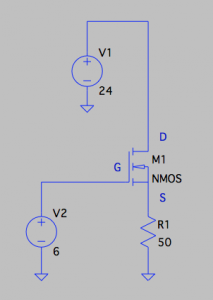But let's think about this. The control voltage is the voltage measured between the gate and the source terminals. When the device is off, the source is basically at ground through the load, so the control voltage, here 6 V, is applied directly. But when the MOSFET is turned fully on it has a very small resistance, it also thus has a very small voltage drop from the power applied to the source terminal (24 V). Thus, the source voltage is effectively the drain voltage. This then requires applying a voltage that is equal to the power being switched PLUS the threshold voltage, which in this example would be something like 30 V.

And indeed, if you try this, you can see that when the gate voltage is switched on, a little bit of current starts to flow, but it is nowhere near the 24 V / 50 Ω = 480 mA that is desired in the example, in fact it's about 40 mA.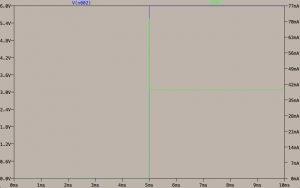The easier way to do this would be to switch the current on the return side. Here, the power supply is applied to the load directly, with the negative of the load attached to the drain terminal and the source terminal connected directly to ground. Again, when the MOSFET is fully on, the resistance and thus voltage drop across the device is very low. This connects the load negative to ground. Also, the gate voltage is now measured relative to ground instead of the supply voltage, and only needs to be a few volts to turn the device on.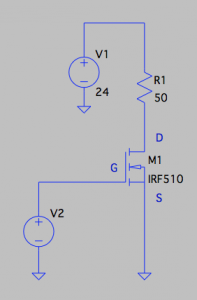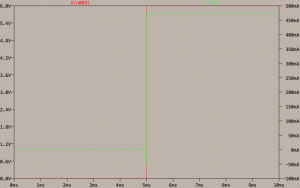Now when the gate voltage switches on, the current through the resistor is nearly 480 mA, as desired.

One other thing to keep in mind is how much power the MOSFET is having to dissipate. In the off state, no current is flowing, so of course there is no power to worry about. Once the threshold voltage is reached, the resistance is starting to decrease rapidly, but also current is starting to flow. In this region, the power consumed by the device can be quite high. Once the device is fully on, the resistance is very low, so the power dissipation drops again.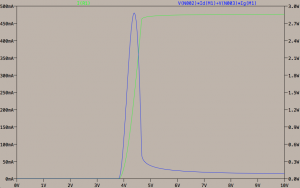This becomes very important if you are doing PWM or high-frequency switching through the MOSFET. One other characteristic of these devices is that the gate is essentially a capacitor. The parameter CISS, the gate capacitance, becomes important in these cases. Recall that resistor/capacitor circuits have a time constant which is how long it takes to reach 63.2% of the applied voltage. If you are switching the device on and off repeatedly, you want to spend as little time in that high power consumption state to allow maximum current handling. Thus, you need to ensure that enough current is available to quickly charge the capacitance.

#### You may also like...

This site uses Akismet to reduce spam. Learn how your comment data is processed.